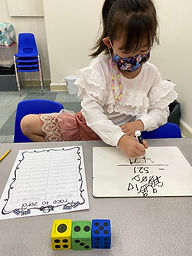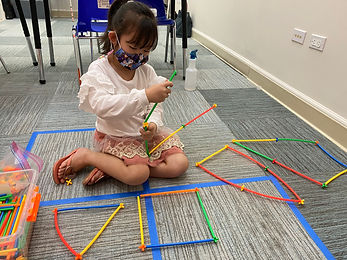Ms. Brianne

Target 1​

Lesson Type:

Continuation

Number Operation

:

Computation

Subtract within 1,000 (regrouping limited to only one place-value).

1:

Understand why the standard algorithm for subtraction is based on place-value.

2:

Understand that when subtracting a multi-digit number, each place-value is being subtracted individually to create the new number (ones are subtracted from ones, tens are subtracted from tens, etc.)

3:

Understand that when adding a multi-digit number, to solve start in the lowest place-value and go left.

4:

Understand how to regroup based on place-value understandings (i.e., ten ones can be composed into one ten – ten tens can be composed into one hundred).

2nd

Vocabulary:

Add, Subtract, Regroup, Ones, Tens, Hundreds

Activities:

• Students practiced solving subtraction and addition problems with regrouping.
• Students played a race to zero game starting with 1,000 and continually subtracting the numbers they roll until they get to zero.Home Exploration

Guiding Questions:Absent Students:

Target 2

:

1:

Classify shapes based on side measurements (2D figures).

2:

Classify shapes as regular (all sides of equal measure).

3:

Classify shapes as irregular (all sides are not of equal measure).

4th

Vocabulary:

Regular, Irregular, Shape, 2D, Side, Angle

Activities:

• Students learned the characteristics of regular and irregular shapes.
• Students sorted pictures of different 2D shapes by whether they are regular or irregular shapes and explained why.
• Students used straws and connectors to build regular and irregular shapes.Home Exploration

Guiding Questions:Target 3

:

Vocabulary:

Activities:Home Exploration